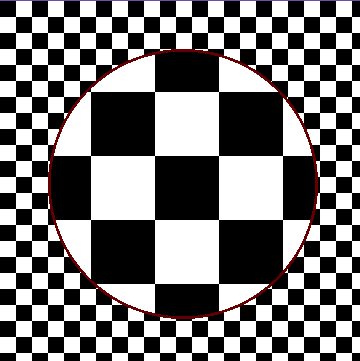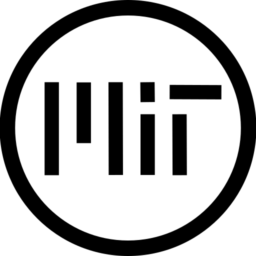A Godot shader that magnifies what’s behind it. Written in Godot Engine v3.4.4.

This shader reads from screen texture. So if the image you want to zoom in isn’t rendered properly, you may end up with an unwanted result.

In order to get this shader working in Godot, you must attach this to a node with a texture. It might work with nodes without textures too, but it’s not tested yet.

``````/*
This is a magnifier shader written in Godot Shading Language which is similar to GLSL ES 3.0.

In order to get this shader working, you must attach this to a node with a texture.
It might work with nodes without textures too, but isn't tested yet.

Author is Yavuz Burak Yalçın @orbbloff

*/

uniform bool is_object_centered; // Note that this needs to match with the sprite's centered property
uniform float magnification:hint_range(0.0, 400.0) = 2.0;
uniform bool filtering;
uniform bool is_round;
uniform float roundness:hint_range(0.0, 2.0) = 1.0;
uniform float circle_radius:hint_range(0.0, 0.71) = 0.5;
uniform float outline_thickness:hint_range(0.0, 0.1) = 0.01;
uniform vec4 outline_color:hint_color = vec4(0.4, 0.0, 0.0, 1.0);

varying flat vec2 center_pos;

void vertex(){
if(is_object_centered){
center_pos = vec2(0.0, 0.0);
}
else{
center_pos = (1.0 / TEXTURE_PIXEL_SIZE) / 2.0;
}
center_pos = (WORLD_MATRIX * vec4(center_pos, 0.0, 1.0)).xy; // From local space texel coordinates
// to screen space pixel coordinates
}

void fragment(){
vec2 screen_resolution = 1.0 / SCREEN_PIXEL_SIZE;
vec2 uv_distance = vec2(0.5) - UV; // UV distance between fragment and object center in local space
vec2 pixel_distance;               // Pixel distance between fragment and object center
pixel_distance.x = center_pos.x - FRAGCOORD.x;
pixel_distance.y = center_pos.y - (screen_resolution.y - FRAGCOORD.y); // Since y component of FRAGCOORD built-in is
// inverted it is extracted from screen resolution
vec2 obj_size = pixel_distance / uv_distance; // Ratio of pixel distance to uv distance gives the objects dimensions
vec2 ratio = obj_size / screen_resolution;    // This gives the ratio of object to screen
float magnify_value = (magnification - 1.0) / magnification; // Maps the magnification value to range[0.0, 1.0)
// while magnification is higher than 1.0
if(is_round){
magnify_value /= smoothstep(0.0, 1.0, length(UV - vec2(0.5))) * roundness + 1.0; // It slightly reduces the magnification
// of points that are far to the center
}

vec2 local_mapped_uv = mix(UV, vec2(0.5 /*center*/), magnify_value); // Calculates a local UV position towards
// the center, proportional to magnification
vec2 difference = local_mapped_uv - UV;
vec2 global_mapped_uv; // Calculates a global UV position to from screen texture
global_mapped_uv.x = SCREEN_UV.x + difference.x * ratio.x;
global_mapped_uv.y = SCREEN_UV.y - difference.y * ratio.y;

if(filtering){
// Applies filter while reading from screen texture
COLOR = texture(SCREEN_TEXTURE, global_mapped_uv);
}
else{
// Doesn't apply filter.
// Since texelFetch function uses screen space pixel coordinates, global_mapped_uv is transformed to pixel coordinates.
COLOR = texelFetch(SCREEN_TEXTURE, ivec2(int(global_mapped_uv.x * screen_resolution.x), int((global_mapped_uv.y) * screen_resolution.y)), 0);
}
// Creates outline
if(length(UV - vec2(0.5)) > circle_radius - outline_thickness){
COLOR = vec4(0.0); // Makes fragments transparent
COLOR = outline_color;
}
}
}``````
###### Tags
magnifier, magnify, scale, zoomThe shader code and all code snippets in this post are under MIT license and can be used freely. Images and videos, and assets depicted in those, do not fall under this license. For more info, see our License terms.